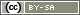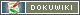# random_p()

`int random_p(array distribution)`

Returns a number from the distribution. Each number can be assigned an individual probability in the process.

• distribution
An array which specifies the possible numbers as keys, and their probability as a value. Therefore, each number is assigned a probability (number `=>` probability). The probability can be specified as a percentage (e.g. `0.25`), or as a relative proportion (e.g. `20`).

Note: The distribution of the drawn values only converges once there have been many draws (n > 1000) of the distribution specified. If you are working with a lower number of cases, use randomization with urns, and enter some numbers more often in the urn, if these should be drawn more often.

Note: Use the function `put()` to save the drawn value in the data record.

## Example 1

In the first example, a number between 1 and 4 is drawn. Numbers 1 and 3 each have a 20% chance of being drawn, number 2 has a 50% chance, and number 4 has a 10% chance. All three random numbers in the first example have the same random distribution.

```// Define distribution
\$distribution = array(
1 => 20,
2 => 50,
3 => 20,
4 => 10
);
// Draw number between 1 and 4
\$number = random_p(\$distribution);
// Save the result of the random draw
put('IV01_01', \$number);
// Show corresponding stimulus
if (\$number == 1) {
text('stimulusA');
} elseif (\$number == 2) {
text('stimulusB');
} elseif (\$number == 3) {
text('stimulusC');
} else {
text('stimulusD');
}```

The probabilities can also be listed as floating-point numbers (with a point as the decimal separator). The numbers can be written directly one after the other as well.

```// Probabilities as floating-point numbers
\$distribution = array(1=>0.2, 2=>0.5, 3=>0.2, 4=>0.1);```

The probabilities do not have to add up to 1 or 100.

```// Probabilities as ratios
\$distribution = array(1=>4, 2=>10, 3=>4, 4=>2);```

And, of course, the distribution does not have to be saved separately in a variable.

```// Draw number between 1 and 4
\$number = random_p(array(
1 => 2,
2 => 5,
3 => 2,
4 => 1
));```

## Example 2

The second example demonstrates how a random selection can be made depending on the respondent's response.

The example assumes that in question “PS19”, how often 7 television stations were watched was requested, ranging from 1 (never), 2 (rarely), 3 (often) and 4 (almost daily).

One of the television stations watched at least rarely should now be selected at random. However, preference should be given to television stations 2, 5 and 6.

```// Create a list in which all television stations watched appear
\$watched = getItems('PS19', 'min', 2);
// If no station is watched, the survey is over
if (count(\$watched) < 1) {
goToPage('end');
}
// Define probabilities for the various stations
\$pAll = array(
1 => 10, 2 => 20, 3 => 10, 4 => 10,
5 => 20, 6 => 30, 7 => 10
);
// A distribution must now be created for the stations watched
\$pWatched = array();
foreach (\$watched as \$station) {
\$pWatched[\$station] = \$pAll[\$station];
}
// Weighted random selection from the stations watched
\$focus = random_p(\$pWatched);
// Store the subject matter
put('PS20_01', \$focus);```